# Applying the Runge-Kutta Method to Second-Order Initial Value Problems

Problem

You need to numerically solve a second-order differential equation of the form: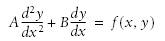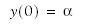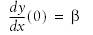Solution

This is a standard initial value problem, and you can implement any of a number of standard numerical integration techniques to solve it using Excel and VBA.

Discussion

Consider this equation and initial conditions: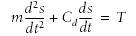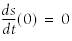Physically, this equation represents the equation of motion for an object moving under some applied thrust, T.[*] Here, m represents the mass of the object, Cd represents a drag factor, and s represents the displacement (position) of the object. This equation allows us to compute the motion of this object.

[*] This problem was adapted from an example I used in one of my previous books, Physics for Game Developers (O'Reilly).

To solve this equation, it helps to rewrite it in the form of two first-order equations. Let v = ds/dt represent the velocity of the object. Now we have the following: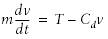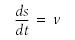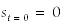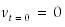These equations represent two coupled first-order equations, and you can apply any of a number of numerical integration schemes to solve them both.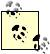From physics, you know that acceleration is the time rate of change of velocity, a = dv/dt. Therefore, you can see that the equation written in terms of velocity is nothing more than an expression of Newton's second law of motion, which states that the sum of all forces acting on an object equals the object's mass times its acceleration.

I decided to use the Runge-Kutta method for this example. This method is based on taking more terms in the Taylor series expansion of a function, as I explained in Recipe 11.1. The caveat here is that you need to apply further Taylor series expansions to estimate the higher-order derivatives. The Runge-Kutta approach reduces the truncation error to something on the order of (dt)5, as opposed to (dt)2 in the case of Euler's basic method (dt is the step size). This means you can usually use larger step sizes while still maintaining accuracy. The trade-off here is more computations per step. But ultimately, with the larger step size you should be able to reduce the overall computational burden using this method.

The Runge-Kutta integration formulas are a little more complicated than the simple formula we used with Euler's basic method. The general equations are as follows: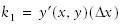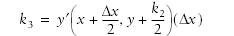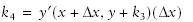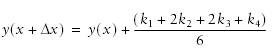y' represents dy/dx in these equations. You can see that every step requires four intermediate computations prior to predicting the next y-value, that is, y(x + dx).

Example 11-3 shows the VBA subroutine I wrote implementing these formulas.

Example 11-3. Implementation of Runge-Kutta method

 ```Public Sub DoRK2ndOrder( ) Dim T As Double ' Thrust Dim Cd As Double ' Drag coefficient Dim M As Double ' Mass Dim dt As Double ' Time step size Dim F As Double ' Force Dim A As Double ' Acceleration Dim Vn As Double ' Velocity at time t Dim Vn1 As Double ' Velocity at time t + dt Dim Sn As Double ' Displacement at time t Dim Sn1 As Double ' Displacement at time t + dt Dim time As Double ' Total time Dim k1 As Double ' Runge-Kutta k1 Dim k2 As Double ' Runge-Kutta k2 Dim k3 As Double ' Runge-Kutta k3 Dim k4 As Double ' Runge-Kutta k4 Dim n As Integer ' Counter controlling total number of time steps Dim C As Integer ' Counter controlling output of results to spreadsheet Dim k As Integer ' Counter controlling output row Dim r As Integer ' Number of output rows   ' Extract given data from the active spreadsheet: With ActiveSheet dt = .Range("dt") T = .Range("T") M = .Range("M") Cd = .Range("Cd") n = .Range("n") r = .Range("r_") End With   ' Initialize variables: k = 1 time = 0 C = n / r Vn = 0 Sn = 0   ' Start iterations: For i = 1 To n   ' Compute k1: F = (T - (Cd * Vn)) A = F / M k1 = dt * A   ' Compute k2: F = (T - (Cd * (Vn + k1 / 2))) A = F / M k2 = dt * A   ' Compute k3: F = (T - (Cd * (Vn + k2 / 2))) A = F / M k3 = dt * A   ' Compute k4: F = (T - (Cd * (Vn + k3))) A = F / M k4 = dt * A   ' Compute velocity at t + dt: Vn1 = Vn + (k1 + 2 * k2 + 2 * k3 + k4) / 6   ' Compute displacement at t + dt using Euler prediction: Sn1 = Sn + Vn1 * dt   ' Update variables: time = time + dt Vn = Vn1 Sn = Sn1   ' Output results to the active spreadsheet: If C >= n / r Then ActiveSheet.Cells(k + 1, 1) = time ActiveSheet.Cells(k + 1, 2) = Sn ActiveSheet.Cells(k + 1, 3) = Vn k = k + 1 C = 0 Else C = C + 1 End If Next i End Sub ```

This is a relatively long subroutine compared to the one shown in Example 11-2 implementing Euler's method, so I commented this one to help you navigate through it.

As usual, several local variables are declared at the beginning of the subroutine. There are a bunch of them here, so I labeled each one in Example 11-3 to indicate their respective purposes.

After the variables are declared, several of them are populated with data retrieved from the active spreadsheet. The spreadsheet is shown in Figure 11-4.

Figure 11-4. Solution using the Runge-Kutta method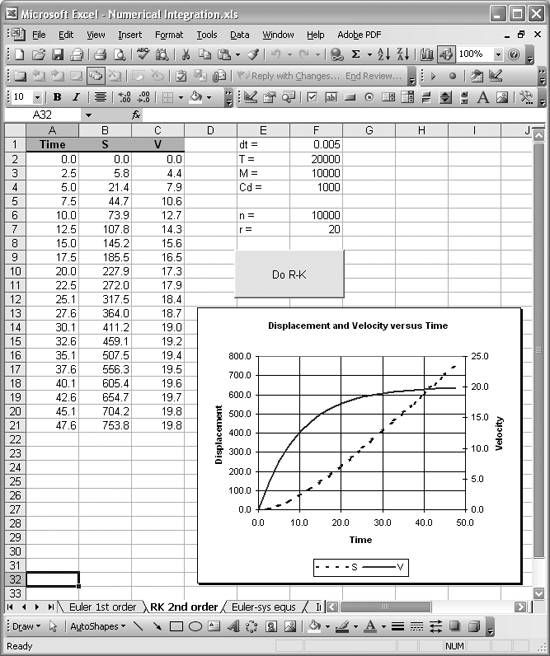Values retrieved from the spreadsheet include the time-step size, thrust, mass, drag coefficient, number of iterations, and number of output rows. The block of code in Example 11-3 containing the With statement performs the data retrieval. Notice that I use cell names to refer to the spreadsheet cells containing the data of interest.See the discussion of Example 9-1 in Recipe 9.3 for information on the use of With statements. Also, take a look at Recipe 1.14 to learn how to define cell names.

The next little section of code simply initializes a few variables. This is where the initial values for displacement and velocity specified in the problem are set.

Next, the For loop iterates computing estimates for displacement and velocity at each step. Taking a closer look at the code within the For loop, you can see how I applied the Runge-Kutta formulas to compute vt+dt. To make things a little more intuitive (at least to me), I broke the computations of the k-values into a few steps, using the analogy of Newton's second law of motion. F in these computations stands for the sum of forces. For this problem, the sum of forces is composed of the thrust force and the drag force. A then represents the object's acceleration, which is equal to the net force divided by the object's mass. Finally, the k-values are computed by multiplying the acceleration by the time step.

I find it easier to think in terms of physical values like force and acceleration whenever possible. Knowing that acceleration is equal to dv/dt, you can readily see that acceleration corresponds to y' in the Runge-Kutta formulas for this particular problem. This is because we broke the original equation up and are dealing with the first one involving velocity.

After all of the k-values are obtained, vt+dt is estimated using the Runge-Kutta formula discussed earlier. Next, a simple Euler estimate is made for the corresponding displacement, st+dt.

Finally, the results are sent to the active spreadsheet in the same manner I showed you in Recipe 11.1. Figure 11-4 shows the results, including a plot of velocity and displacement versus time, using a time step of 0.005. You should play around with this time step to see how the accuracy of the results is affected. (If this were a class, I'd assign that exercise for homework.)

I should also mention that I used a button control, as you can see in Figure 11-4, to call the subroutine when the button is clicked. Refer back to Recipe 9.3 to learn how to add this sort of button control and assign its functionality.Excel Scientific and Engineering Cookbook (Cookbooks (OReilly))
ISBN: 0596008791
EAN: 2147483647
Year: N/A
Pages: 206
Authors: David M Bourg